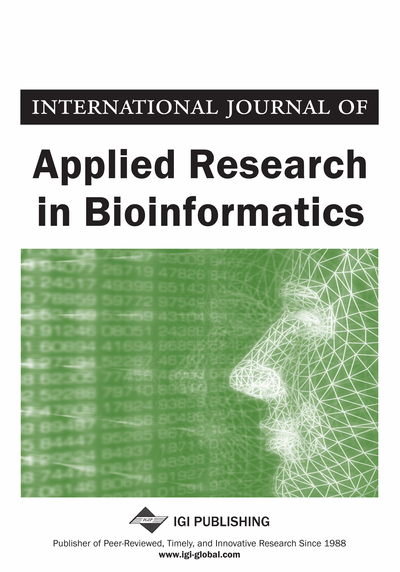# About Mathematical Model-Based Geothermal Water Resources in Jermuk Region in Armenia

Sedrak Sedrakyan (Urartu University, Yerevan, Armenia)
DOI: 10.4018/IJARB.2020010103
Available
\$29.50
No Current Special Offers

## Abstract

This study took place in Jermuk region in Armenia. The study site was chosen purposively, and is related to implementation and geothermal policy scenario; hence this study focuses at the central government. The study was done from June to August 2019.
Article Preview
Top

## Introduction

The object studied is government policy. The main object in public policy is the society. The population in this study is all people who are able to assess the energy policy, especially geothermal policy. In this case, the community of Jermuk acts as a representative of a society capable of assessing energy policy especially geothermal energy. The population size in this study was 770 people. The sample is part of the population that can be used to explain (generalize) the population. The sampling technique chosen was the Stratified Proportional Random Sampling, which takes some of the 770 members.

Data analysis used to test the hypothetical model built is Partial Least Square (PLS), employing the SmartPLS package computer program (Solimun et al., 2017). This method is used for the following reasons. First, the analysis model is tiered, and the model of structural equation satisfies the recursive model. Second, measurement is for formative variables, i.e. each variable in this study is measured formatively, considering each indicator is mutually exclusive.

In PLS analysis, there are two models. The first is the model of measurement (outer model), that is measurement of variable from each indicator. There are five (5) measurement models in this study according to the number of research variables, with the equation of measurement model with formative indicators, as follows (λ is the measurement model coefficient / outer weight, and e is the measurement model error):

X1 = λ11 X1.1 + λ12 X1.213 X1.3 + e1X2 = λ21 X2.1 + λ22 X2.223 X2.3 + e2M = λ31 M1 + λ32 M233 M334 M4 + e3Y1 = λ41 Y1.1 + λ42 Y1.243 Y1.344 Y1.4 + e4Y2 = λ51 Y2.1 + λ52 Y2.253 Y2.354 Y2.4 + e5

The second is the structural model (inner model), i.e. testing the relationship between variables (Solimun et al., 2017). The structural model in this study is as follows (γ is the coefficient of structural model or inner weight, and e is a structural model error):

Y1 = γ11 X1 + γ21 X2 + γ31 M*X1 + γ41 M*X2 + u1Y2 = γ12 X1 + γ22 X2 + γ32 M*X1 + γ42 M*X2 + γ52 Y1 + γ62 M*Y1 + u2

## Complete Article List

Search this Journal:
Reset
Volume 13: 1 Issue (2023): Forthcoming, Available for Pre-Order
Volume 12: 2 Issues (2022): 1 Released, 1 Forthcoming
Volume 11: 2 Issues (2021)
Volume 10: 2 Issues (2020)
Volume 9: 2 Issues (2019)
View Complete Journal Contents Listing